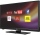# Fraction calculator

The calculator performs basic and advanced operations with fractions, expressions with fractions combined with integers, decimals, and mixed numbers. It also shows detailed step-by-step information about the fraction calculation procedure. Solve problems with two, three, or more fractions and numbers in one expression.

## Result:

### 23/4 + 12/5 = 83/20 = 4 3/20 = 4.15

Spelled result in words is eighty-three twentieths (or four and three twentieths).

### How do you solve fractions step by step?

1. Conversion a mixed number 2 3/4 to a improper fraction: 2 3/4 = 2 3/4 = 2 · 4 + 3/4 = 8 + 3/4 = 11/4

To find new numerator:
a) Multiply the whole number 2 by the denominator 4. Whole number 2 equally 2 * 4/4 = 8/4
b) Add the answer from previous step 8 to the numerator 3. New numerator is 8 + 3 = 11
c) Write a previous answer (new numerator 11) over the denominator 4.

Two and three quarters is eleven quarters
2. Conversion a mixed number 1 2/5 to a improper fraction: 1 2/5 = 1 2/5 = 1 · 5 + 2/5 = 5 + 2/5 = 7/5

To find new numerator:
a) Multiply the whole number 1 by the denominator 5. Whole number 1 equally 1 * 5/5 = 5/5
b) Add the answer from previous step 5 to the numerator 2. New numerator is 5 + 2 = 7
c) Write a previous answer (new numerator 7) over the denominator 5.

One and two fifths is seven fifths
3. Add: 11/4 + 7/5 = 11 · 5/4 · 5 + 7 · 4/5 · 4 = 55/20 + 28/20 = 55 + 28/20 = 83/20
For adding, subtracting, and comparing fractions, it is suitable to adjust both fractions to a common (equal, identical) denominator. The common denominator you can calculate as the least common multiple of both denominators - LCM(4, 5) = 20. In practice, it is enough to find the common denominator (not necessarily the lowest) by multiplying the denominators: 4 × 5 = 20. In the next intermediate step, the fraction result cannot be further simplified by canceling.
In words - eleven quarters plus seven fifths = eighty-three twentieths.

#### Rules for expressions with fractions:

Fractions - use the slash “/” between the numerator and denominator, i.e., for five-hundredths, enter 5/100. If you are using mixed numbers, be sure to leave a single space between the whole and fraction part.
The slash separates the numerator (number above a fraction line) and denominator (number below).

Mixed numerals (mixed fractions or mixed numbers) write as non-zero integer separated by one space and fraction i.e., 1 2/3 (having the same sign). An example of a negative mixed fraction: -5 1/2.
Because slash is both signs for fraction line and division, we recommended use colon (:) as the operator of division fractions i.e., 1/2 : 3.

Decimals (decimal numbers) enter with a decimal point . and they are automatically converted to fractions - i.e. 1.45.

The colon : and slash / is the symbol of division. Can be used to divide mixed numbers 1 2/3 : 4 3/8 or can be used for write complex fractions i.e. 1/2 : 1/3.
An asterisk * or × is the symbol for multiplication.
Plus + is addition, minus sign - is subtraction and ()[] is mathematical parentheses.
The exponentiation/power symbol is ^ - for example: (7/8-4/5)^2 = (7/8-4/5)2

#### Examples:

subtracting fractions: 2/3 - 1/2
multiplying fractions: 7/8 * 3/9
dividing Fractions: 1/2 : 3/4
exponentiation of fraction: 3/5^3
fractional exponents: 16 ^ 1/2
adding fractions and mixed numbers: 8/5 + 6 2/7
dividing integer and fraction: 5 ÷ 1/2
complex fractions: 5/8 : 2 2/3
decimal to fraction: 0.625
Fraction to Decimal: 1/4
Fraction to Percent: 1/8 %
comparing fractions: 1/4 2/3
multiplying a fraction by a whole number: 6 * 3/4
square root of a fraction: sqrt(1/16)
reducing or simplifying the fraction (simplification) - dividing the numerator and denominator of a fraction by the same non-zero number - equivalent fraction: 4/22
expression with brackets: 1/3 * (1/2 - 3 3/8)
compound fraction: 3/4 of 5/7
fractions multiple: 2/3 of 3/5
divide to find the quotient: 3/5 ÷ 2/3

The calculator follows well-known rules for order of operations. The most common mnemonics for remembering this order of operations are:
PEMDAS - Parentheses, Exponents, Multiplication, Division, Addition, Subtraction.
BEDMAS - Brackets, Exponents, Division, Multiplication, Addition, Subtraction
BODMAS - Brackets, Of or Order, Division, Multiplication, Addition, Subtraction.
GEMDAS - Grouping Symbols - brackets (){}, Exponents, Multiplication, Division, Addition, Subtraction.
Be careful, always do multiplication and division before addition and subtraction. Some operators (+ and -) and (* and /) has the same priority and then must evaluate from left to right.

## Fractions in word problems:3 3/4 + 2 3/5 + 5 1/2 Show your solution.Why does 1 3/4 + 2 9/10 equal 4.65? How do you solve this?2 and 1/8th plus 1 and 1/3rd =Add two mixed fractions: 2 4/6 + 1 3/6Add this two mixed numbers: 1 5/6 + 2 2/11=What is 4 1/2+2/7-213/14?To three-eighths of one third, we add five quarters of one half and multiply the sum by four. How much will we get?Ann ate a third of a pizza and then another quater. Total part of pizza eaten by Ann and how much pizza is left?7 is added to the sum of 4/5 and 6/7How many hours the Andersons watched TV in all Wednesday 3/1 hr Thursdays 2/3 hr Friday 4/5 hr Saturday 3/4 hrWhat is the sum of 2/3+3/5?Find a fraction equivalent to the recurring decimal? 0.435643564356Evaluate expression using BODMAS rule: 1 1/4+1 1/5÷3/5-5/8